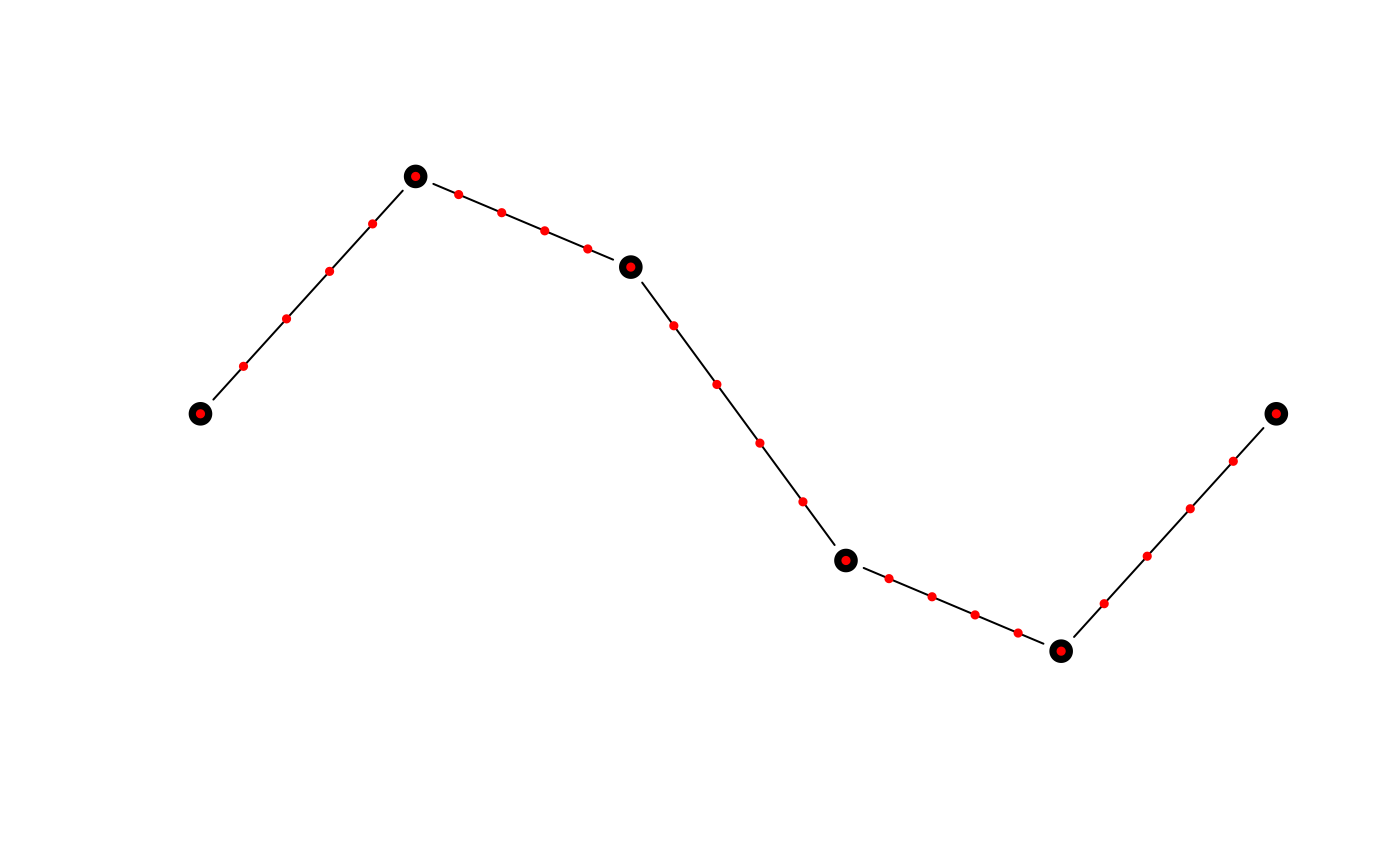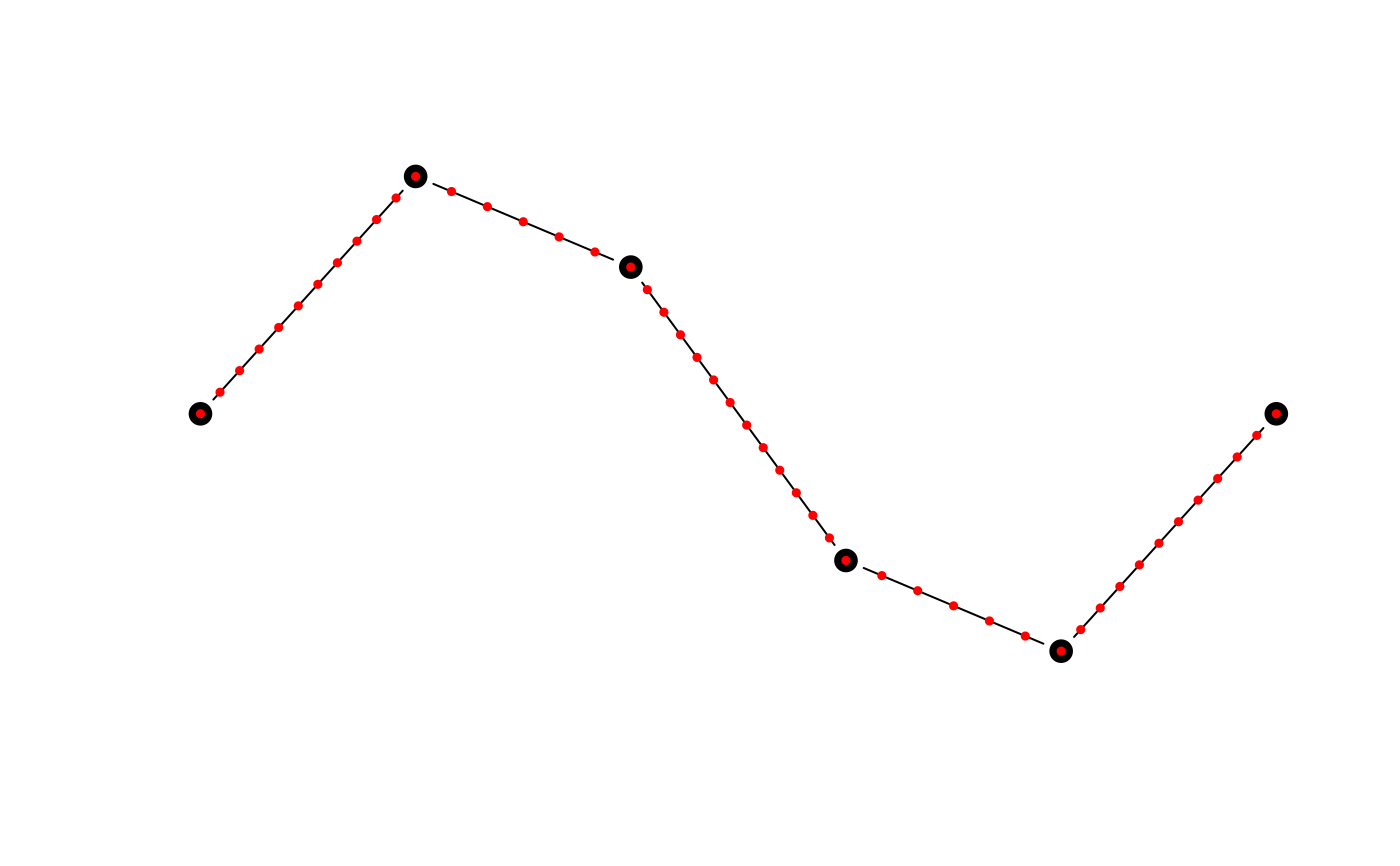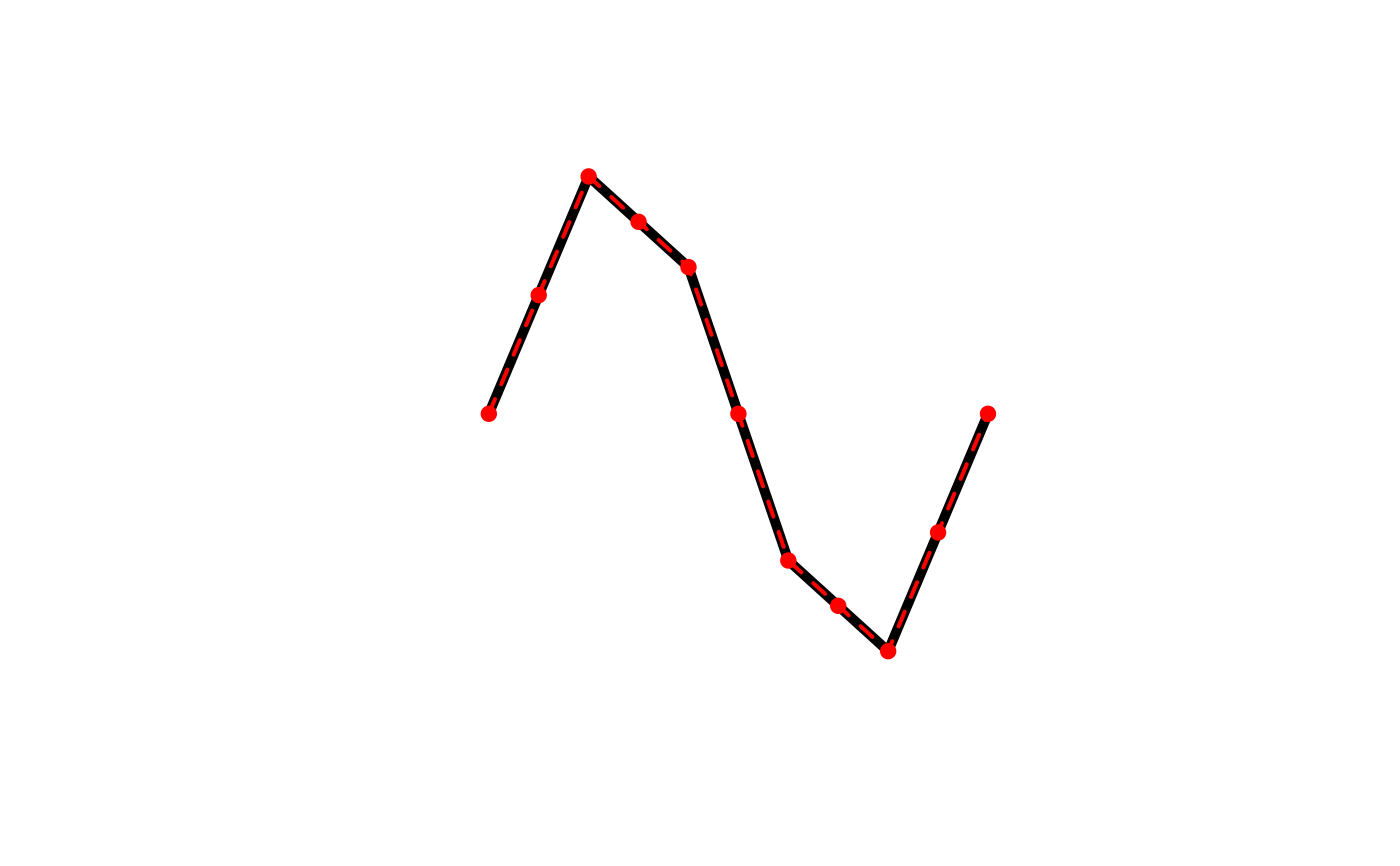This function adds additional vertices to lines or polygons via linear interpolation, always while keeping the original vertices. Each line segment will be split into equal length sub-segments. This densification algorithm treats all vertices as Euclidean points, i.e. new points will not fall on a great circle between existing vertices, rather they'll be along a straight line.

smooth_densify(x, wrap = FALSE, n = 10L, max_distance)

## Arguments

x numeric matrix; matrix of coordinates. logical; whether the coordinates should be wrapped at the ends, as for polygons and closed lines, to ensure a smooth edge. integer; number of times to split each line segment. Ignored if max_distance is specified. numeric; the maximum distance between vertices in the resulting matrix. This is the Euclidean distance and not the great circle distance.

## Value

A matrix with the coordinates of the densified curve.

## Details

This function works on matrices of points and is generally not called directly. Instead, use smooth() with method = "densify" to apply this smoothing algorithm to spatial features.

## Examples

# smooth_densify works on matrices of coordinates
# use the matrix of coordinates defining a line as an example
m <- jagged_lines$geometry[][] m_dense <- smooth_densify(m, n = 5) class(m) #>  "matrix" "array" class(m_dense) #>  "matrix" "array" plot(m, type = "b", pch = 19, cex = 1.5, axes = FALSE, xlab = NA, ylab = NA) points(m_dense, col = "red", pch = 19, cex = 0.5)# max_distance can be used to ensure vertices are at most a given dist apart m_md <- smooth_densify(m, max_distance = 0.05) plot(m, type = "b", pch = 19, cex = 1.5, axes = FALSE, xlab = NA, ylab = NA) points(m_md, col = "red", pch = 19, cex = 0.5)# smooth is a wrapper for smooth_densify that works on spatial features library(sf) l <- jagged_lines$geometry[]
l_dense <- smooth(l, method = "densify", n = 2)
class(l)
#>  "XY"         "LINESTRING" "sfg"       class(l_dense)
#>  "XY"         "LINESTRING" "sfg"       plot(l, lwd = 5)
plot(l_dense, col = "red", lwd = 2, lty = 2, add = TRUE)
plot(l_dense %>% st_cast("MULTIPOINT"), col = "red", pch = 19,